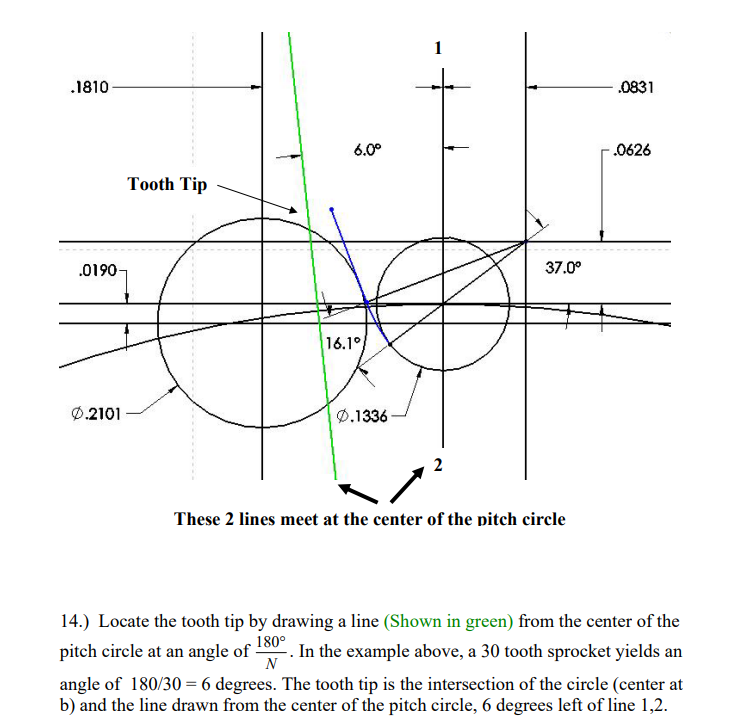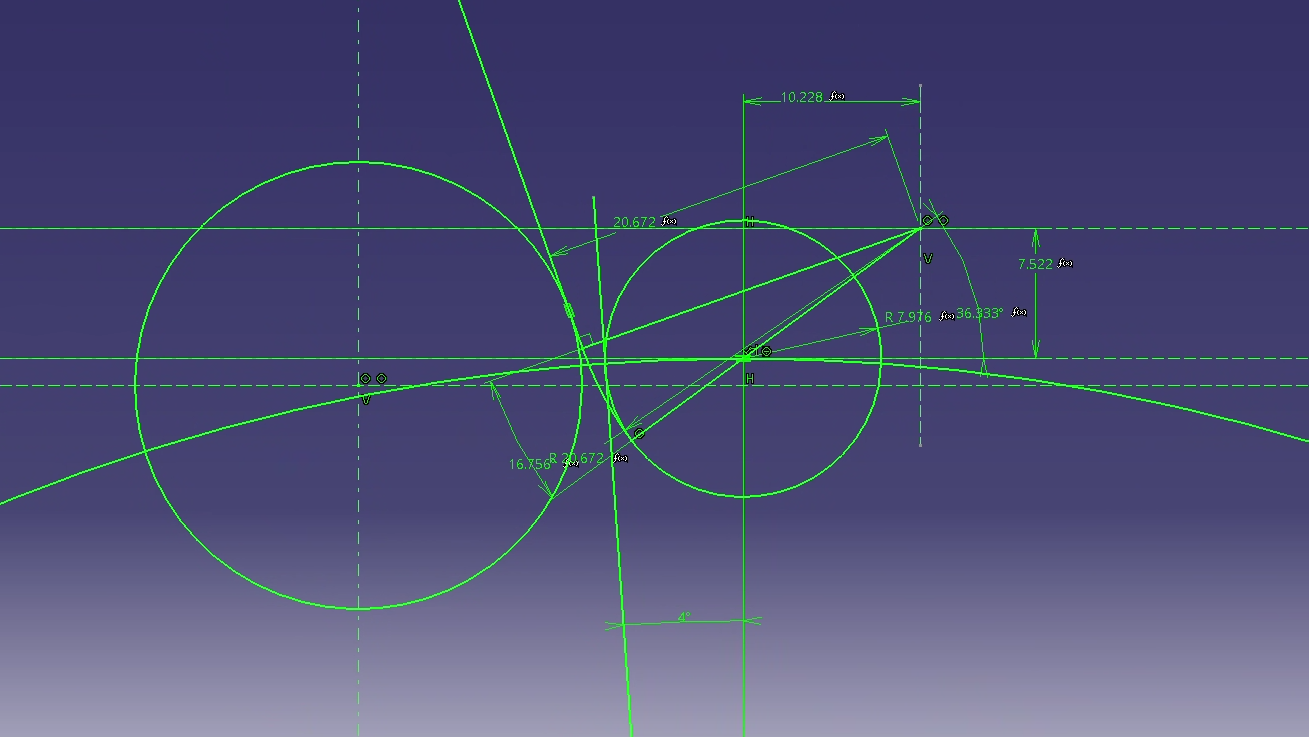# Gears

### Created by Simourv on 9 March, 2018

#### Sproket Design Problem

Hi everyone,

I am having an issue modelling a sprocket using equations I have found from an example that originated from a textbook (American Chain Association Chains for Power Transmission and Material Handling handbook). The example (http://www.gearseds.com/files/design_draw_sprocket_5.pdf) walks through the steps and explains every point well however I have come across an issue that I am not quite understanding.this section defines the tooth tip and it is based on a function 180/N where N = number of teeth. This works for the example perfectly fine however any other examples I try this logic doesn't work. The example I made used metric values I had gotten from a paper (https://www.researchgate.net/publication/341968409_Designing_and_Validation_of_a_Sprocket_Wheel_for_a_Formula_Student_Vehicle) however when I reach this same point in the design process using the values from the paper the design breaks.If this angle is set to 4 degrees which are based on the calculation of 180/45 where 45 is the number of teeth the angle of 4 is too small to produce the tooth geometry.

Am I doing something wrong?